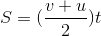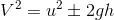# Physics: Equations of Motion, derivation, Questions and solutions

In the last article, The definition and types of Motion were discussed. Now this is an article to better understand Equations of motion with several questions solved.

Related Terms

• Displacement: It is the distance moved in a specified direction. It is a vector quantity.
• Distance: It is the magnitude of separation between two points. It is a scalar quantity.
• Speed: It is the rate of change of distance with time. It is a scalar quantity.
• Velocity: It is the rate of change of displacement with time. It is a vector quantity.
READ MORE: On mass and weight, scalar and vector quantities.
• Acceleration: It is the increasing rate of change of velocity with time. It is a vector quantity.
• Retardation: It is the decreasing rate of change of velocity with time.

Equations of motion

1)2)3)L 4)Derivation

Average speed = s/t

(V+u)/2 =s/t

where, V =final velocity
U = Initial velocity
S = Distance
T = time
Make "s" subject of the formula...........(1)

Average acceleration= change in Velocity/time

a = (v-u)/t

at = (v-u)

V = U + at ...........(2)

Substitute (2) in (1)

S =[ (u + at +u)/2]t.

S =[(2u+at)/2]t........(3)
From (2)

V = u + at
t =(v-u)/a

Substitute the value of t in (1)

S = (v+u)/2 x (v-u)/a.....(4)

Question 1:
A car travels with a uniform velocity of 30m/s for 5s and then comes to a rest in the next 10s with a uniform deceleration.
Find,
a) deceleration
b) total distance travelled.

Solution,
a) using, V = u + at
Deceleration= -a
0 = 30m/s - a(10)
10a = 30
a = 3m/s/s

b) Let, "Stage A" be the period before          deceleration.
"Stage B" be the period after deceleration.

At Stage A,

U= 30m/s
t1= 5s
S1= Distance travelled at stage A  ?
Using,
From the V-T graph,
Initial Velocity (U) = S1/T1

S1 = ( 30 x 5) m

S1 = 150 m

At Stage B,

U= 30 m/s
V = 0
T2 = 10s
S2 = Distance travelled at stage B ?

Using,0 = (30)(30) + 2(-3)(s2)

900 = 6(s2)

S2 =150m

Total distance travelled (S) = S1 + S2

S = (150 + 150) m/s

S = 300m.

Question 2:
A bus moves from rest with an initial acceleration of 2m/s^2 for the first 10 s. It then accelerates at a uniform rate of 1m/s^2 for another 15s. It continues at a constant speed for 70s and finally comes to a rest in 20s by a uniform deceleration. From the graph calculate.
I)Total distance travelled
II) Average speed for the journey
III) Average retardation as it is brought to rest.
IV) Maximum speed attained by the motion.

Solution,

From the V-T graph

I).  for the first 10s,
U= 0

a = 2m/s/s

t1 = 10s

Velocity (V)= u + at

V = 0 + (2)(10)
V = 20m/s
Distance (S1) = [(v +u)/2] t

S1 = [ (20 +0)/2] x 10

S1 = [10 x 10] = 100m

For the second 15s
U= 20m/s

t2 = 15s
Velocity (v)  = ( 20 + 15) m/s
V = 35 m/s

Distance (S2) = [(v +u)/2] t

S2 = [ (35 +20)/2] x 10

S2 = [41.25 x 10] = 412.5m

For the third 70s,
V = constant at 35m/s
t3 = 70s

V =  S3/t3

S3 = (35 x 70) m

S3 = 2450m

For the last 20s (Retardation or deceleration).

V = 0

U = 35m/s

t4 = 20s

S4 = [(v +u)/2] t4

S2 = [ (0 +35)/2] x 20

S2 = [35 x 10] = 350m

Total distance travelled(S)= S1 + S2 + S3 + S4

S = [ 100 + 412.5 + 2450 + 350]

S = 3312.5m

II) Average velocity =  S / T

T = [ 10 + 15 + 70 + 20] s

T = 115s

V = 3312.5/115 = 28.8 m/s

Average velocity spent for the journey = 28.8m/s

III) Average Retardation = (v-u)/t4

V = 0
U = 35m/s
AR = (0 - 35)/20
AR = 1.75 m/ s (square)

Average Retardation as it's brought to rest = 1.75m/s^2

Motion under gravity
Motion under the influence of gravity is based on acceleration due to gravity(g)  of a free falling object and  +/- g sign conventions.

FormulaQuestion 1
A cricket ball is thrown vertically upwards with an initial velocity of 40m/s . Find,
I) it's velocity after 3s
II) Maximum height attained and the time it
III) The total time taken for the ball to return to the ground again.

Solution,

I) V = u - gt
V = 40 - 10 (3)
V = 40 -30 = 10m/s

II) using,V = 0
U = 40m/s
g = 10m/s/s
From formula,

(40)(40) = 2 (10) h

1600 = 20 h

h =80 mTherefore,
80 = 40t -  5t^2 ( it is now a quadratic equation)

16 =  8t - t^2

t^2 - 8t + 16 = 0

(t - 4)(t - 4) = 0

t = 4s